RADIUS AND ARC DIMENSIONS

When the ends or corners of an object are to be rounded, a radius, arc, or curve is shown and is dimensioned by one of several methods, as shown in Figure 4.8. The methods of dimensioning include the use of an angu­lar dimension and its radius (Figure 4.8a); two linear dimensions that indicate where the arc terminates, and its radius (Figure 4.8b); and a radius and centerlines that indicate the scope of the arc by inspection (Figure 4.8b). In the case of an arc with multiple radii, the dimensions shown are the location dimensions for the radii and the size of the radii, Figure 4.8(c). Note that the letter “R” is used to signify radius and is located preced­ing the dimension. In cases where a diameter dimension is applied, the symbol 0 is used and precedes the dimension.

For dimensioning rounds, fillets, and roundouts the radius of the arc with a leader is usually sufficient, Figure 4.9(b).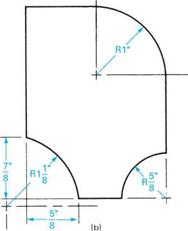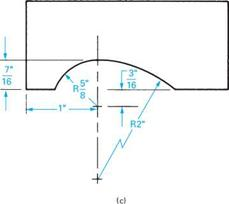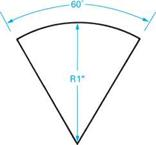FIGURE 4.8 ■ Dimensioning arcs and radii.

Other variations in dimensioning radii and arcs are illustrated in Figure 4.9.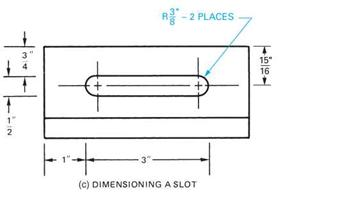SYMBOL FOR ARC, SIGNIFYING THE LINEAR DIMENSION APPLIES TO THE LENGTH OF THE ARC AND NOT TO ITS CHORDAL SIZE

 (a) APPLYING A LINEAR DIMENSION TO AN ARCSR .750 (SR SIGNIFIES SPHERICAL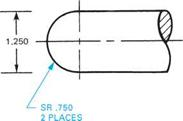R1 " (ROUND)

 R1 " (ROUNDOUT)

 (FILLET)

 1 ..

 R T(d) DIMENSIONING A SPHERICAL RADIUS

 (b) DIMENSIONING A ROUND, FILLET, AND ROUNDOUT

FIGURE 4.9 ■ Variations in dimensioning arcs and radii.